# Tessellation

﻿
TessellationA tessellation of pavement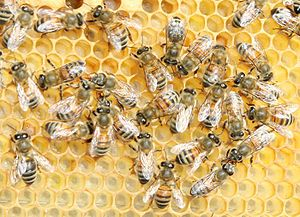A honeycomb is an example of a tessellated natural structure

A tessellation or tiling of the plane is a pattern of plane figures that fills the plane with no overlaps and no gaps. One may also speak of tessellations of parts of the plane or of other surfaces. Generalizations to higher dimensions are also possible. Tessellations frequently appeared in the art of M. C. Escher, who was inspired by studying the Moorish use of symmetry in the Alhambra tiles during a visit in 1922. Tessellations are seen throughout art history, from ancient architecture to modern art.

In Latin, tessella is a small cubical piece of clay, stone or glass used to make mosaics. The word "tessella" means "small square" (from "tessera", square, which in its turn is from the Greek word for "four"). It corresponds with the everyday term tiling which refers to applications of tessellations, often made of glazed clay.

## History

In 1619 Johannes Kepler did one of the first documented studies of tessellations when he wrote about the regular and semiregular tessellation, which are coverings of a plane with regular polygons. Some two hundred years later in 1891, the Russian crystallographer Yevgraf Fyodorov proved that every periodic tiling of the plane features one of seventeen different groups of isometries. Fedorov's work marked the unofficial beginning of the mathematical study of tessellations. Other prominent contributors include Shubnikov and Belov (1951); and Heinrich Heesch and Otto Kienzle (1963).

## Wallpaper groups

Tilings with translational symmetry can be categorized by wallpaper groups, of which 17 exist. All seventeen of these groups are represented in the Alhambra palace in Granada, Spain. Of the three regular tilings two are in the p6m wallpaper group and one is in p4m.

## Tessellations and color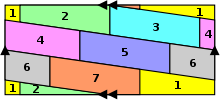If this parallelogram pattern is colored before tiling it over a plane, seven colors are required to ensure each complete parallelogram has a consistent color that is distinct from that of adjacent areas. (This tiling can be compared to the surface of a torus.) Coloring after tiling, only four colors are needed.

When discussing a tiling that is displayed in colors, to avoid ambiguity one needs to specify whether the colors are part of the tiling or just part of its illustration. See also symmetry.

The four color theorem states that for every tessellation of a normal Euclidean plane, with a set of four available colors, each tile can be colored in one color such that no tiles of equal color meet at a curve of positive length. Note that the coloring guaranteed by the four-color theorem will not in general respect the symmetries of the tessellation. To produce a coloring which does, as many as seven colors may be needed, as in the picture at right.

Copies of an arbitrary quadrilateral can form a tessellation with 2-fold rotational centers at the midpoints of all sides, and translational symmetry whose basis vectors are the diagonal of the quadrilateral or, equivalently, one of these and the sum or difference of the two. For an asymmetric quadrilateral this tiling belongs to wallpaper group p2. As fundamental domain we have the quadrilateral. Equivalently, we can construct a parallelogram subtended by a minimal set of translation vectors, starting from a rotational center. We can divide this by one diagonal, and take one half (a triangle) as fundamental domain. Such a triangle has the same area as the quadrilateral and can be constructed from it by cutting and pasting.

## Regular and semi-regular tessellations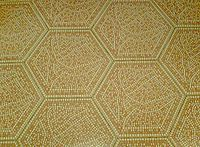Hexagonal tessellation of a floor

A regular tessellation is a highly symmetric tessellation made up of congruent regular polygons. Only three regular tessellations exist: those made up of equilateral triangles, squares, or hexagons. A semiregular tessellation uses a variety of regular polygons; there are eight of these. The arrangement of polygons at every vertex point is identical. An edge-to-edge tessellation is even less regular: the only requirement is that adjacent tiles only share full sides, i.e. no tile shares a partial side with any other tile. Other types of tessellations exist, depending on types of figures and types of pattern. There are regular versus irregular, periodic versus nonperiodic, symmetric versus asymmetric, and fractal tessellations, as well as other classifications.

Penrose tilings using two different polygons are the most famous example of tessellations that create aperiodic patterns. They belong to a general class of aperiodic tilings that can be constructed out of self-replicating sets of polygons by using recursion.

A monohedral tiling is a tessellation in which all tiles are congruent. Spiral monohedral tilings include the Voderberg tiling discovered by Hans Voderberg in 1936, whose unit tile is a nonconvex enneagon; and the Hirschhorn tiling discovered by Michael Hirschhorn in the 1970s, whose unit tile is an irregular pentagon.

## Self-dual tessellations

Tilings and honeycombs can also be self-dual. All n-dimensional hypercubic honeycombs with Schlafli symbols {4,3n−2,4}, are self-dual.## Tessellations and computer models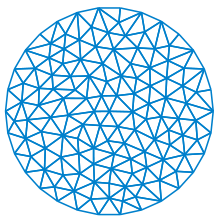A tessellation of a disk used to solve a finite element problem.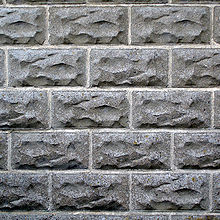These rectangular bricks are connected in a tessellation which, considered as an edge-to-edge tiling, is topologically identical to a hexagonal tiling; each hexagon is flattened into a rectangle whose long edges are divided in two by the neighboring bricks.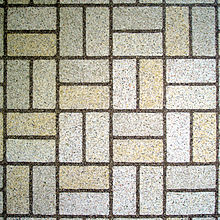This basketweave tiling is topologically identical to the Cairo pentagonal tiling, with one side of each rectangle counted as two edges, divided by a vertex on the two neighboring rectangles.

In the subject of computer graphics, tessellation techniques are often used to manage datasets of polygons and divide them into suitable structures for rendering. Normally, at least for real-time rendering, the data is tessellated into triangles, which is sometimes referred to as triangulation. Tessellation is a staple feature of DirectX 11 and OpenGL.

In computer-aided design the constructed design is represented by a boundary representation topological model, where analytical 3D surfaces and curves, limited to faces and edges constitute a continuous boundary of a 3D body. Arbitrary 3D bodies are often too complicated to analyze directly. So they are approximated (tessellated) with a mesh of small, easy-to-analyze pieces of 3D volume — usually either irregular tetrahedrons, or irregular hexahedrons. The mesh is used for finite element analysis.

Mesh of a surface is usually generated per individual faces and edges (approximated to polylines) so that original limit vertices are included into mesh. To ensure approximation of the original surface suits needs of the further processing 3 basic parameters are usually defined for the surface mesh generator:

• Maximum allowed distance between planar approximation polygon and the surface (aka "sag"). This parameter ensures that mesh is similar enough to the original analytical surface (or the polyline is similar to the original curve).
• Maximum allowed size of the approximation polygon (for triangulations it can be maximum allowed length of triangle sides). This parameter ensures enough detail for further analysis.
• Maximum allowed angle between two adjacent approximation polygons (on the same face). This parameter ensures that even very small humps or hollows that can have significant effect to analysis will not disappear in mesh.

Algorithm generating mesh is driven by the parameters. Some computer analyses require adaptive mesh, which is made finer (using stronger parameters) in regions where the analysis needs more detail.

Some geodesic domes are designed by tessellating the sphere with triangles that are as close to equilateral triangles as possible.

## Tessellations in nature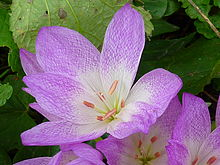Tessellate pattern in a Colchicum flower

Basaltic lava flows often display columnar jointing as a result of contraction forces causing cracks as the lava cools. The extensive crack networks that develop often produce hexagonal columns of lava. One example of such an array of columns is the Giant's Causeway in Northern Ireland.

The Tessellated pavement in Tasmania is a rare sedimentary rock formation where the rock has fractured into rectangular blocks.

Within botany, the term "tessellate" describes a checkered pattern, for example on a flower petal, tree bark, or fruit.

## Number of sides of a polygon versus number of sides at a vertex

For an infinite tiling, let a be the average number of sides of a polygon, and b the average number of sides meeting at a vertex. Then (a − 2)(b − 2) = 4. For example, we have the combinations (3, 6), (3 1  3 , 5), (3 3  4 , 4 2  7 ), (4, 4), (6, 3), for the tilings in the article Tilings of regular polygons.

A continuation of a side in a straight line beyond a vertex is counted as a separate side. For example, the bricks in the picture are considered hexagons, and we have combination (6, 3). Similarly, for the basketweave tiling often found on bathroom floors, we have (5, 3 1  3 ).

For a tiling which repeats itself, one can take the averages over the repeating part. In the general case the averages are taken as the limits for a region expanding to the whole plane. In cases like an infinite row of tiles, or tiles getting smaller and smaller outwardly, the outside is not negligible and should also be counted as a tile while taking the limit. In extreme cases the limits may not exist, or depend on how the region is expanded to infinity.

For finite tessellations and polyhedra we have$( a - 2 ) ( b - 2 ) = 4 \left( 1 - \frac{\chi}{F} \right) \left( 1 - \frac{\chi}{V} \right)$

where F is the number of faces and V the number of vertices, and χ is the Euler characteristic (for the plane and for a polyhedron without holes: 2), and, again, in the plane the outside counts as a face.

The formula follows observing that the number of sides of a face, summed over all faces, gives twice the total number of sides in the entire tessellation, which can be expressed in terms of the number of faces and the number of vertices. Similarly the number of sides at a vertex, summed over all vertices, also gives twice the total number of sides. From the two results the formula readily follows.

In most cases the number of sides of a face is the same as the number of vertices of a face, and the number of sides meeting at a vertex is the same as the number of faces meeting at a vertex. However, in a case like two square faces touching at a corner, the number of sides of the outer face is 8, so if the number of vertices is counted the common corner has to be counted twice. Similarly the number of sides meeting at that corner is 4, so if the number of faces at that corner is counted the face meeting the corner twice has to be counted twice.

A tile with a hole, filled with one or more other tiles, is not permissible, because the network of all sides inside and outside is disconnected. However it is allowed with a cut so that the tile with the hole touches itself. For counting the number of sides of this tile, the cut should be counted twice.

For the Platonic solids we get round numbers, because we take the average over equal numbers: for (a − 2)(b − 2) we get 1, 2, and 3.

From the formula for a finite polyhedron we see that in the case that while expanding to an infinite polyhedron the number of holes (each contributing −2 to the Euler characteristic) grows proportionally with the number of faces and the number of vertices, the limit of (a − 2)(b − 2) is larger than 4. For example, consider one layer of cubes, extending in two directions, with one of every 2 × 2 cubes removed. This has combination (4, 5), with (a − 2)(b − 2) = 6 = 4(1 + 2 / 10)(1 + 2 / 8), corresponding to having 10 faces and 8 vertices per hole.

Note that the result does not depend on the edges being line segments and the faces being parts of planes: mathematical rigor to deal with pathological cases aside, they can also be curves and curved surfaces.

## Tessellations of other spaces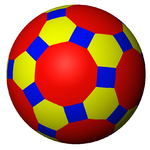An example tessellation of the surface of a sphere by a truncated icosidodecahedron.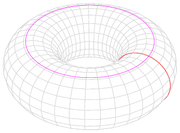A torus can be tiled by a repeating matrix of isogonal quadrilaterals.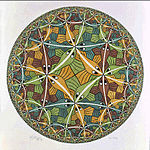M.C.Escher, Circle Limit III (1959)

As well as tessellating the 2-dimensional Euclidean plane, it is also possible to tessellate other n-dimensional spaces by filling them with n-dimensional polytopes. Tessellations of other spaces are often referred to as honeycombs. Examples of tessellations of other spaces include:

• Tessellations of n-dimensional Euclidean space. For example, 3-dimensional Euclidean space can be filled with cubes to create the cubic honeycomb.
• Tessellations of n-dimensional elliptic space, either the n-sphere (spherical tiling, spherical polyhedron) or n-dimensional real projective space (elliptic tiling, projective polyhedron).
For example, projecting the edges of a regular dodecahedron onto its circumsphere creates a tessellation of the 2-dimensional sphere with regular spherical pentagons, while taking the quotient by the antipodal map yields the hemi-dodecahedron, a tiling of the projective plane.
• Tessellations of n-dimensional hyperbolic space. For example, M. C. Escher's Circle Limit III depicts a tessellation of the hyperbolic plane (using the Poincaré disk model) with congruent fish-like shapes. The hyperbolic plane admits a tessellation with regular p-gons meeting in q's whenever$\tfrac{1}{p}+\tfrac{1}{q} < \tfrac{1}{2}$; Circle Limit III may be understood as a tiling of octagons meeting in threes, with all sides replaced with jagged lines and each octagon then cut into four fish.

See (Magnus 1974) for further non-Euclidean examples.

There are also abstract polyhedra which do not correspond to a tessellation of a manifold because they are not locally spherical (locally Euclidean, like a manifold), such as the 11-cell and the 57-cell. These can be seen as tilings of more general spaces.

Wikimedia Foundation. 2010.

### Look at other dictionaries:

• Tessellation — Tes sel*la tion, n. The act of tessellating; also, the mosaic work so formed. J. Forsyth. [1913 Webster] …   The Collaborative International Dictionary of English

• tessellation — 1650s, from L.L. tessellatus (see TESSELLATED (Cf. tessellated)) …   Etymology dictionary

• tessellation — noun a) The property or fact of tessellating. Squares can be used for tessellation. b) A tiling pattern with no gaps; the result of tessellating an area or plane. This is a tessellation of the plane with squares and regular octagons. See Also:… …   Wiktionary

• Tessellation — In der Mathematik wird mit einer Parkettierung der (euklidischen) Ebene, auch als Pflasterung, Belegung, Zerlegung, Kachelung oder Tessellation bezeichnet, eine lückenlose und überlappungsfreie Überdeckung der Ebene durch Parkettsteine… …   Deutsch Wikipedia

• tessellation — tessellate ► VERB 1) (often as adj. tessellated) decorate (a floor) with mosaics. 2) Mathematics cover (a plane surface) by repeated use of a single shape. DERIVATIVES tessellation noun. ORIGIN Latin tessellare, from tessera …   English terms dictionary

• tessellation — noun Date: 1660 1. a. mosaic b. a covering of an infinite geometric plane without gaps or overlaps by congruent plane figures of one type or a few types 2. an act of tessellating ; the state of being tessellated …   New Collegiate Dictionary

• tessellation — /tes euh lay sheuhn/, n. 1. the art or practice of tessellating. 2. tessellated form or arrangement. 3. tessellated work. Also, tesselation. [1650 60; TESSELLATE (V.) + ION] * * * …   Universalium

• tessellation — tesÂ·selÂ·laÂ·tion || â€štesÉª leÉªÊƒn n. filling with mosaics …   English contemporary dictionary

• tessellation — tes·sel·la·tion …   English syllables

• tessellation — /tɛsəˈleɪʃən/ (say tesuh layshuhn) noun 1. the act or art of tessellating. 2. tessellated form or arrangement. 3. tessellated work …   Australian English dictionary